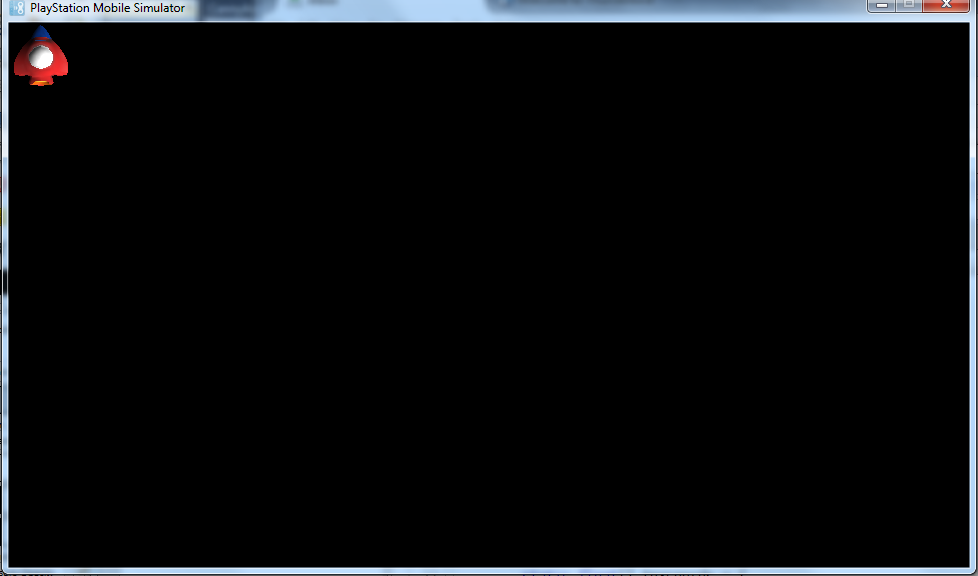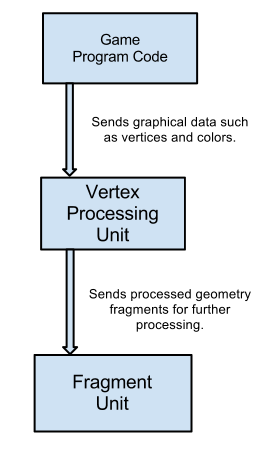# PSM Tutorial #2-1 : Shader Programs

## 2012-12-05 2:36 AM

We’ll start out by breaking down sony example project for drawing an image on screen. Once we understand what’s going on we can move on to making our own sprite rendering system which will be a lot more flexible.

Let’s take a look at the code then go through it section by section like the previous tutorial. You can also open this code up and run it. It’s located in the “Tutorial\Sample02_01” of your samples folder for PSM. Here is the code:

``````public class AppMain
{
static protected GraphicsContext graphics;
static Texture2D texture;

static float[] vertices=new float;

static float[] texcoords = {
0.0f, 0.0f,// 0 top left.
0.0f, 1.0f,// 1 bottom left.
1.0f, 0.0f,// 2 top right.
1.0f, 1.0f,// 3 bottom right.
};

static float[] colors = {
1.0f,1.0f,1.0f,1.0f,// 0 top left.
1.0f,1.0f,1.0f,1.0f,// 1 bottom left.
1.0f,1.0f,1.0f,1.0f,// 2 top right.
1.0f,1.0f,1.0f,1.0f,// 3 bottom right.
};

const int indexSize = 4;
static ushort[] indices;

static VertexBuffer vertexBuffer;

// Width of texture.
static float Width;

// Height of texture.
static float Height;

static Matrix4 unitScreenMatrix;

public static void Main (string[] args)
{
Initialize ();

while (true) {
SystemEvents.CheckEvents ();
Update ();
Render ();
}
}

public static void Initialize ()
{
graphics = new GraphicsContext();
ImageRect rectScreen = graphics.Screen.Rectangle;

texture = new Texture2D("/Application/resources/Player.png", false);

Width = texture.Width;
Height = texture.Height;

vertices=0.0f;// x0
vertices=0.0f;// y0
vertices=0.0f;// z0

vertices=0.0f;// x1
vertices=1.0f;// y1
vertices=0.0f;// z1

vertices=1.0f;// x2
vertices=0.0f;// y2
vertices=0.0f;// z2

vertices=1.0f;// x3
vertices=1.0f;// y3
vertices=0.0f;// z3

indices = new ushort[indexSize];
indices = 0;
indices = 1;
indices = 2;
indices = 3;

//vertex pos,               texture,       color
vertexBuffer = new VertexBuffer(4, indexSize, VertexFormat.Float3, VertexFormat.Float2, VertexFormat.Float4);

vertexBuffer.SetVertices(0, vertices);
vertexBuffer.SetVertices(1, texcoords);
vertexBuffer.SetVertices(2, colors);

vertexBuffer.SetIndices(indices);
graphics.SetVertexBuffer(0, vertexBuffer);

unitScreenMatrix = new Matrix4(
Width*2.0f/rectScreen.Width,0.0f,    0.0f, 0.0f,
0.0f,   Height*(-2.0f)/rectScreen.Height,0.0f, 0.0f,
0.0f,   0.0f, 1.0f, 0.0f,
-1.0f,  1.0f, 0.0f, 1.0f
);

}

public static void Update ()
{

}

public static void Render ()
{
graphics.Clear();

graphics.SetTexture(0, texture);

graphics.DrawArrays(DrawMode.TriangleStrip, 0, indexSize);

graphics.SwapBuffers();
}
}

``````

Here is what it looks like when you run the code:A lot more things to look at for this tutorial. We’ll be taking a look at what OpenGL is really doing. There’s a lot of concepts that I’ll touch on but are quite deep so I will provide some supplementary reading for those who want to further their knowledge on the various topics we touch on.

Let’s start by looking over the declarations:

``````public class AppMain
{
static protected GraphicsContext graphics;
static Texture2D texture;

static float[] vertices=new float;

static float[] texcoords = {
0.0f, 0.0f,// 0 top left.
0.0f, 1.0f,// 1 bottom left.
1.0f, 0.0f,// 2 top right.
1.0f, 1.0f,// 3 bottom right.
};

static float[] colors = {
1.0f,1.0f,1.0f,1.0f,// 0 top left.
1.0f,1.0f,1.0f,1.0f,// 1 bottom left.
1.0f,1.0f,1.0f,1.0f,// 2 top right.
1.0f,1.0f,1.0f,1.0f,// 3 bottom right.
};

const int indexSize = 4;
static ushort[] indices;

static VertexBuffer vertexBuffer;

// Width of texture.
static float Width;

// Height of texture.
static float Height;

static Matrix4 unitScreenMatrix;

``````

We already know what a graphics context is from the http://levelism.com/psp-vita-opengl-tutorial-1-part-1-explaining-the-code-in-a-new-project/ so let’s move on to the ShaderProgram class:

``````static ShaderProgram shaderProgram;

``````

A shader program is a class that encapsulates shader code. A shader is a set of code that instructs the GPU chip on how to handle graphics data you send it from your program. The way this works is first you prepare your data in your programming code, then you send it to the Vertex processing unit of the GPU. The vertex shader program is run once for every vertex that you send to the vertex processing unit.This is where the geometric functions are performed and data is prepared to be handed to the next step of the process, the fragment unit.

The fragment unit takes the vertex data and then draws every fragment onto the screen. A fragment is a pixel on a piece of geometry. This is where you perform pixel operations. If you have ever seen the cool filters and color changing stuff you can do in a image editing program such as photoshop or GIMP this is where you would perform similar effects.

The vertex step of the process can be used for many useful things. One example would be transforming all your vertex data. For instance one of the most common uses is if you have a large world that is stored in a array of vertices that you send to the vertex unit and then send it some mathematical information that explains where a camera is inside your world, the vertex processing unit will use this camera data to move the entire world so that when it renders the world is positioned according to the camera information. Essentially what this means is the actual geometric math required to have a camera is performed on the vertex processing unit.

Here is a very very simple vertex shader.

``````void main(float4 in a_Position    : POSITION,
float4 out v_Position   : POSITION,
uniform float4x4 u_WorldMatrix)
{
v_Position = mul(a_Position, u_WorldMatrix);
}

``````

What we have here in the main function parameters is a in variable which represents the current vertex we have sent the vertex processing unit.

The word “in” marks the data as incoming data from the program code. Next we have an out variable which will represent the transformed vertex data that we sent to the next stage of the GPU process (the fragment shader). The word “out” says that this data will be sent to the fragment shader. In the case of vertex data the out parameter doesn’t directly send the data to the fragment shader. There are internal processes which determine whether or not the data reaches the fragment shader by calculating it’s depth from the camera and whether or not it is actually in the space viewable by the camera. We will discuss this more in depth in a later tutorial when we deal with more advanced fragment operations. For the most part other data such as colors and texture coordinates are sent directly to the fragment shader.

Finally we have a uniform matrix variable. Uniform means a piece of data in our programming code that is marked to come into the vertex shader. Uniforms are how we pass information we need from the game code to our vertex shader. In this case you can think of the uniform matrix as our camera we discussed earlier. Finally we have the line:

``````v_Position = mul(a_Position, u_WorldMatrix);

``````

What this does is performs matrix multiplication to calculate the new position of the vertex based on the input camera data. Matrix and vector math operations are part of shader language math operations so luckily we do not have to have full knowledge of how they work. Essentially if you multiply the vertex against the “camera transformation matrix” you have a vertex that is now in the correct position relative to the camera.

Some other examples of cool things you can do on the vertex processing unit include things like skeletal character animation and model deformations. While it’s true you could do some of these operations in your programming code it’s almost always advisable to do this on the vertex processing unit because it’s a specialized processor made specifically to do geometric operations, you will almost always have much better performance doing these operations on the GPU rather than the CPU.

Once the vertex processing unit is finished processing its data it is sent to the fragment unit (also knows and the pixel shader). As stated before a fragment is a pixel on a piece of geometry. The fragment unit program is run once for every fragment. At it’s most basic level you just receive an incoming fragment and then send it back to the screen with a color. In reality depending on what you are doing you might be sampling a texture for a 3d model or performing a cool filter to give the game a artistic impressionist effect or toon style. Here is an example of an very simple fragment shader.

``````void main(float4 out color      : COLOR)
{
color =  float4(0, 1.0, 0, 1.0);
}

``````

As you can see here we have one out parameter which is the color of the pixel we will be rendering to the screen. Usually we would have some information coming in from the vertex shader but in the case of simplicity we will just color the incoming fragment green and paint it to the screen. The coloring of the fragment green is done on this line:

``````color =  float4(0, 1.0, 0, 1.0);

``````

The color out variable is assigned to be a float4. Float4’s are an array of 4 floating point variables. In this case we interpret it as a color structure with the format RGBA which stands for Red,Green,Blue,Alpha. The RGB portion should be fairly self-explanatory. The alpha represents how transparent the pixel is. 0 is fully transparent and 1 is fully opaque. So in our case we have R=0, G=1.0, B=0 and A=1.0. So this is why we would get a fragment that is green and fully opaque.

So to reiterate the process of how graphics get drawn to the screen I’ve written this small schematic to reinforce the concept.In the next part of this tutorial we will look at the shaders included with this example which are slightly more complex. It will be a shorter part. Hopefully you have the knowledge of how the graphics system works now. If you have any feedback, questions or comments leave a comment in on this post and I will do my best to address them.

I would also like to thank Bruce Sutherland for reviewing this tutorial and making some corrections in regards to how vertex out parameters in the vertex shader are not directly sent to the fragment shader. Here is his explanation on what happens to a vertex out parameter from the vertex processing unit:

The output of the vertex shader is still a vertex in 3D space.

In OpenGL and DirectX the vertices are mapped to what’s called the canonical view volume. In OpenGL that’s a cube which goes from -1, -1, -1 to 1, 1, 1.

You then have another stage in the GPU which carries out clipping and then screen mapping. These stages are carried out in Geometry Shaders on newer versions of DirectX and OpenGL on the desktop but haven’t made it to mobile gpus yet.

Some people can get confused by vertex shaders when they don’t realise what the output they are trying to generate actually is.

Extra vertex data like texture co-ordinates would mostly go untouched, except for clipped vertices, the texture co-ord sent to the fragment shader would probably be whatever the GPU calculates the text coord to be at the point of intersection.Bruce also has a blog with tutorials and topics on programming including Android related topics if you are interested in that. You can visit his site here:

http://brucesutherland.blogspot.com.au/

Tags: psm_tutorial